Select Page

Are you afraid that AI might take your job? Make sure you are the one who is building it.

STAY RELEVANT IN THE RISING AI INDUSTRY! 🖖

Elon Musk made it clear that he doesn’t like Facebook, however, Tesla still uses one piece of technology that comes from Zukenberg’s laboratory. We can bet that it made its way into SpaceX as well and that it was an important part of the recent launch too. This technology has a big advocate in the AI world and that is Yan LeCunn, father of modern Convolutional Neural Networks. That is correct, we are talking about PyTorch. This deep learning framework is extremely popular among the research community and we can witness that in the past couple of years it gained popularity in the industry as well. In this article, we explore the basic principles of PyTorch and how we can use can be used for simple tasks.Generally speaking PyTorch as a tool has two big goals. The first one is to be NumPy for GPUs. This doesn’t mean that NumPy is a bad tool, it just means that it doesn’t utilize the power of GPUs. The second goal of PyTorch is to be a deep learning framework that provides speed and flexibility. That is why it is so popular in the research community because it provides a platform in which users can quickly perform experiments. Apart from that, building models in PyTorch is very easy.

## Installation

Installing PyTorch is quite easy and you can create a command that you need to run for the installation on this page. All you need to do is enter your environment details and run the command. Here is how I’ve set it up for my machine: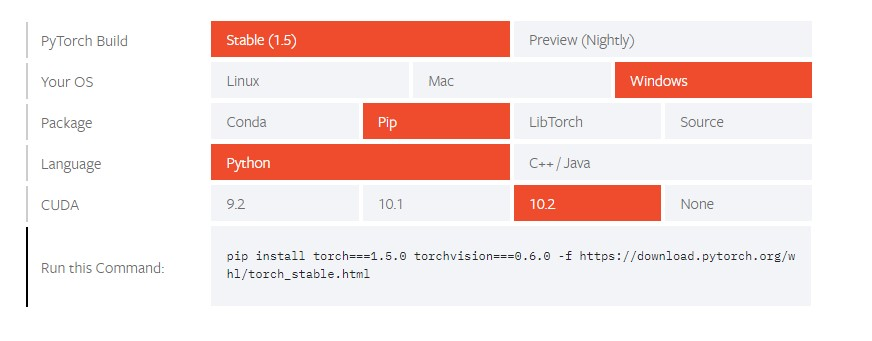To verify that you have installed it correctly, run Jupyter Notebook and try to import torch:

``````import torch

torch.__version__``````
``'1.5.0'``

In general, a lot of concepts in machine learning and deep learning can be abstracted using multi-dimensional matrices – tensors. Mathematically speaking, tensors are described as geometric objects that describe linear relationships between other geometric objects. For the simplification of all concepts, tensors can be observed as multi-dimensional arrays of data. When we observe them like n-dimensional arrays we can apply matrix operations easily and effectively. That is what PyTorch is actually doing. In this framework, a tensor is a primitive unit used to model scalars, vectors and matrices located in the central class of the package torch.Tensor. We can do various operations with tensors, but first, let’s see how we can create and initialize them.

### Creating and Initializing Tensors

Here is how we can define scalar, vector, and matrix:

``````# Scalar
tensor_1 = torch.tensor(11.)
print('------ Scalar ------')
print(tensor_1)
print(tensor_1.dtype)
print(tensor_1.shape)
print('\n')

# Vector
tensor_2 = torch.tensor([11, 23, 9, 33])
print('------ Vector ------')
print(tensor_2)
print(tensor_2.dtype)
print(tensor_2.shape)
print('\n')

# Matrix
tensor_3 = torch.tensor([[5., 6],
[7, 4],
[9, 11]])

print('------ Matrix ------')
print(tensor_3)
print(tensor_3.dtype)
print(tensor_3.shape)
print('\n')

# 3D Array
tensor_4 = torch.tensor([
[[11, 12, 13],
[13, 14, 15]],
[[15, 16, 17],
[17, 18, 19.]]])

print('------ 3D Array ------')
print(tensor_4)
print(tensor_4.dtype)
print(tensor_4.shape)
print('\n')``````

In the code snippet above, we created scalar, vector, matrix and 3D array. Note that for vector we use integers and for other floats. Also, we printed out their data types and shapes. Here is the output:

``````------ Scalar ------
tensor(11.)
torch.float32
torch.Size([])

------ Vector ------
tensor([11, 23,  9, 33])
torch.int64
torch.Size()

------ Matrix ------
tensor([[ 5.,  6.],
[ 7.,  4.],
[ 9., 11.]])
torch.float32
torch.Size([3, 2])

------ 3D Array ------
tensor([[[11., 12., 13.],
[13., 14., 15.]],

[[15., 16., 17.],
[17., 18., 19.]]])
torch.float32
torch.Size([2, 2, 3])``````As you can see it is quite easy to create all the types of data that we need quickly. There are various tensor initialization functions. For example, you can create uninitialized tensor, or tensor initialized with zeroes, ones or with some random value:

``````# Uninitialized (does not contain definite known values before it is used.)
tensor = torch.empty(2, 3)
print(tensor)

# Tensor with all zeroes
tensor = torch.zeros(2, 3)
print(tensor)

# Tensor with all ones
tensor = torch.ones(2, 3)
print(tensor)

# Tensor with randomly initialized values
tensor = torch.rand(2, 3)
print(tensor)
``````
``````tensor([[1.0286e-38, 9.0919e-39, 9.3674e-39],
[9.2755e-39, 1.4013e-43, 0.0000e+00]])
tensor([[0., 0., 0.],
[0., 0., 0.]])
tensor([[1., 1., 1.],
[1., 1., 1.]])
tensor([[0.1093, 0.3990, 0.5312],
[0.2732, 0.6488, 0.4613]])``````

As we mentioned one of the benefits of using PyTorch is that you can perform NumPy operations in parallel on GPU. This means that we can create tensors from NumPy arrays, and vice-versa create NumPy arrays from PyTorch tensors. The cool thing is that the tensor and NumPy array share underlying memory locations, so the changing one will change the other:

``````import numpy as np

numpy_array = np.array([[1, 2], [3, 4.]])
print(numpy_array)
print('\n')

tensor = torch.from_numpy(numpy_array)
print(tensor)
print('\n')

re_numpy_array = tensor.numpy()
print(re_numpy_array)``````
``````[[1. 2.]
[3. 4.]]

tensor([[1., 2.],
[3., 4.]], dtype=torch.float64)

[[1. 2.]
[3. 4.]]``````

### Tensor Operations

Torch tensors can easily be manipulated and there are various options to perform mathematical operations on them. For example, we can do something like this:

``````x = torch.tensor(3.)
w = torch.tensor(4.)
b = torch.tensor(5.)

res = w*x + b
res``````
``tensor(17.)``There are also the different syntax of these operations that you can use. For example, if you want to add two tensors there are several options that will give the same results:

``````x = torch.rand(2, 3)
y = torch.rand(2, 3)

result1 = x + y

print(result1)
print(result2)``````
``````tensor([[1.8197, 1.0063, 1.8231],
[0.2407, 1.0290, 0.8837]])
tensor([[1.8197, 1.0063, 1.8231],
[0.2407, 1.0290, 0.8837]])``````

Apart from this, you can reshape tensors using view function:

``````tensor = torch.randn(3, 3)
reshaped = tensor.view(9)

print(tensor, tensor.size())
print(reshaped, reshaped.size())``````
``````tensor([[-0.4982,  1.0700,  1.0479],
[-0.2169,  0.0897,  0.2885],
[ 1.3385,  0.0141, -2.3748]]) torch.Size([3, 3])
tensor([-0.4982,  1.0700,  1.0479, -0.2169,  0.0897,  0.2885,  1.3385,  0.0141,
-2.3748]) torch.Size()``````

Finally, it is important to mention that you can index tensors just like you would index NumPy arrays:

``````tensor = torch.randn(3, 3)
print(tensor[:, 1])``````
``tensor([ 1.0461, -0.7462,  0.0028])``

There are many other functions that you can do with PyTorch tensors, but for start, these will get you going.

Another core concept of Pytorch is the notion of gradients. They are located in torch.autograd package. In essence, this package performs automatic differentiation and it is define-by-run. This means that backpropagation is defined by how your code is run. In order to utilize this functionality, all you have to do is set PyTorch tensor attribute `.requires_grad` as `True`. This will signal PyTorch to record all operations performed on that tensor. Once the computation or some interaction is finished, you can call function `.backward()` and have all the gradients computed automatically. The gradient for each tensor is stored into `.grad` attribute of the class. Here is an example. First, let’s create 3×3 tensor:

``````tensor = torch.randn(3, 3)
print(tensor[:, 1])``````
``````tensor([[1., 1., 1.],
[1., 1., 1.],Then let’s add a scalar to it:

``````increased = tensor + 2
print(increased)``````
``````tensor([[3., 3., 3.],
[3., 3., 3.],

Note that grad attribute is now filled and that performed operations are recorded. This means that we can perform some more operations and calculate gradients and print them out:

``````temp = increased * increased * 3
output = temp.mean()
output.backward()

``````tensor([[4., 4., 4.],
[4., 4., 4.],
[4., 4., 4.]])``````

## Linear Regression

Ok, this should be enough PyTorch background to help us implement Linear Regression. Linear Regression is one of the foundational algorithms of machine learning. It attempts to model the relationship between two variables by fitting a linear equation to observed data. In our example, we have multiple variables, which means that we will implement Multiple Linear Regression. Note that we are not following strict definitions here, and we are using some techniques that are more used in deep learning than in standard machine learning. This is because PyTorch is a primarily deep learning framework and in the next several articles we will use it to develop neural networks. Anyhow, Linear Regression can be described by the formula: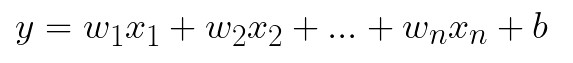Where is the input vector (presented as x1, x2, …, xn) and y is the output target. The goal of Linear regression is to predict correct weights vector w and bias b that will for new values for input x give correct values for output y. Linear regression learns these values during the training process where and x values are known (supervised learning). Here is the dataset that we use for this example: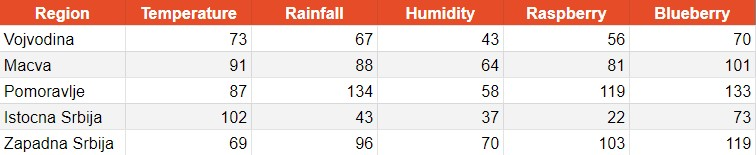So, we have five regions with three input values: the average temperature in Fahrenheit, average rainfall in mm, and average humidity in %. As the output, we have two values, crop yields for raspberry and blueberry. The goal is to create the linear regression model that will from the given data be able to learn to predict correct crop yields. Technically our linear regression formula looks something like this: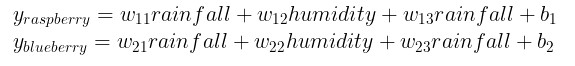Ok, so let’s implement Linear Regression from scratch. First, we need to define input and output data and create PyTorch tensors from them:

``````inputs = np.array([[73, 67, 43],
[91, 88, 64],
[87, 134, 58],
[102, 43, 37],
[69, 96, 70]], dtype='float32')

outputs = np.array([[56, 70],
[81, 101],
[119, 133],
[22, 37],
[103, 119]], dtype='float32')

inputs = torch.from_numpy(inputs)
outputs = torch.from_numpy(outputs)``````

Apart from that, we need to initialize weights and biases:

``````w = torch.randn(2, 3, requires_grad=True)

In this case, our model is simple, it needs to multiply input values with weights and add bias to it.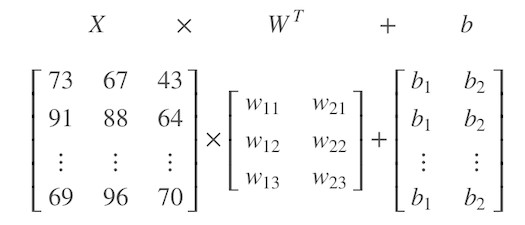We can define model as a function:

``````def model(x):
return x @ w.t() + b``````

Operator @ in PyTorch represents matrix multiplication and t() function on a tensor, returns the transposed value of it. Another thing we need to do is to define the loss function. This function will tell us how well our model performs. There are multiple options that we can pick for this function, but we will use Mean Squared Error function. It does exactly what the name suggests, here is the formula:In essence, this function calculates differences between predicted values and real values, squares them and calculated the average. Here is the implementation:

``````def mse(pred, real):
difference = pred - real

Method `torch.sum` returns the sum of all the elements in a tensor, and the `.numel` method returns the number of elements in a tensor. Finally, we need to decide how to utilize this error in order to modify weights and biases. We do that using optimization technique Gradient Descent. In essence, what we are trying to do is calculate the best values for weights and biases that will minimize the loss. We do so by training our model in several epochs. During every epoch, we will calculate predictions, loss and gradients. Based on the gradients we will adjust the weights by subtracting a small quantity proportional to the gradient. Here is what training for 200 epochs looks like:

``````for i in range(200):
predictions = model(inputs)
loss = mse(predictions, outputs)

print(f'Epoch: {i} - Loss: {loss}')

loss.backward()

Note that everytime we call zero_() method in the end to reset the gradients. The output looks like this:

``````...
Epoch: 187 - Loss: 135.3285675048828
Epoch: 188 - Loss: 134.859375
Epoch: 189 - Loss: 134.3938751220703
Epoch: 190 - Loss: 133.9319305419922
Epoch: 191 - Loss: 133.4735565185547
Epoch: 192 - Loss: 133.01876831054688
Epoch: 193 - Loss: 132.56735229492188
Epoch: 194 - Loss: 132.11936950683594
Epoch: 195 - Loss: 131.6748046875
Epoch: 196 - Loss: 131.23353576660156
Epoch: 197 - Loss: 130.7956085205078
Epoch: 198 - Loss: 130.36087036132812
Epoch: 199 - Loss: 129.929443359375``````

Note how the loss, ie. prediction error is getting smaller and smaller. In the end, we can compare predictions after 200 epochs and real values:

``````predictions = model(inputs)
predictions``````
``````tensor([[ 59.0074,  72.3907],
[ 81.6869,  90.8632],
[116.9137, 151.9167],
[ 31.7110,  47.7871],
``outputs``
``````tensor([[ 56.,  70.],
[ 81., 101.],
[119., 133.],
[ 22.,  37.],
[103., 119.]])``````

Considering that we are performing validation on the training set and that we still have some differences we can say that these results could be better. Anyhow, we managed to get close and in the process learn how to use PyTorch. Now, we can proceed and build more powerful tools like neural networks.## Conclusion

In this article, we had a chance to check out the basics of PyTorch. We also saw how we can implement Linear Regression with it. In the next article, we will go one level higher and use it to implement simple neural networks.

Are you afraid that AI might take your job? Make sure you are the one who is building it.

STAY RELEVANT IN THE RISING AI INDUSTRY! 🖖#### Nikola M. Zivkovic

CAIO at Rubik's Code

Nikola M. Zivkovic a CAIO at Rubik’s Code and the author of book “Deep Learning for Programmers“. He is loves knowledge sharing, and he is experienced speaker. You can find him speaking at meetups, conferences and as a guest lecturer at the University of Novi Sad.

Rubik’s Code is a boutique data science and software service company with more than 10 years of experience in Machine Learning, Artificial Intelligence & Software development. Check out the services we provide.

Ultimate Guide to Machine Learning with Python

Everything from Python basics to the deployment of Machine Learning algorithms to production in one place.

Become a Machine Learning Superhero TODAY!